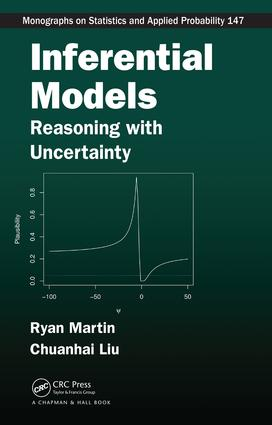Inferential Models

Reasoning with Uncertainty, 1st Edition

Chapman and Hall/CRC

256 pages | 37 B/W Illus.

Purchasing Options:\$ = USD
Hardback: 9781439886489
pub: 2015-09-25
SAVE ~\$19.59
\$97.95
\$78.36
x
eBook (VitalSource) : 9780429109416
pub: 2015-09-25
from \$46.98

FREE Standard Shipping!

Description

A New Approach to Sound Statistical Reasoning

Inferential Models: Reasoning with Uncertainty introduces the authors’ recently developed approach to inference: the inferential model (IM) framework. This logical framework for exact probabilistic inference does not require the user to input prior information. The authors show how an IM produces meaningful prior-free probabilistic inference at a high level.

The book covers the foundational motivations for this new IM approach, the basic theory behind its calibration properties, a number of important applications, and new directions for research. It discusses alternative, meaningful probabilistic interpretations of some common inferential summaries, such as p-values. It also constructs posterior probabilistic inferential summaries without a prior and Bayes’ formula and offers insight on the interesting and challenging problems of conditional and marginal inference.

This book delves into statistical inference at a foundational level, addressing what the goals of statistical inference should be. It explores a new way of thinking compared to existing schools of thought on statistical inference and encourages you to think carefully about the correct approach to scientific inference.

Reviews

"The book . . . delivers on its promise. It should be read by all statisticians with an interest in the foundations and development of the statistical methods for inference."

~Michael J. Lew, University of Melbourne

" . . . the book covers the motivations for the IM framework, the basic theory behind its calibration properties, a number of its applications and gives a new way of thinking compared to existing schools of thought on statistical inference"

~Apostolos Batsidis (Ioannina), Zentralblatt MATH

Preliminaries

Introduction

Assumed background

Scientific inference: An overview

Prediction and inference

Outline of the book

Prior-Free Probabilistic Inference

Introduction

Probabilistic inference

Existing approaches

On the role of probability in statistical inference

Our contribution in this book

Two Fundamental Principles

Introduction

Validity principle

Efficiency principle

Recap

On conditioning for improved efficiency

Inferential Models

Introduction

Basic overview

Inferential models

Theoretical validity of IMs

Theoretical optimality of IMs

Two more examples

Concluding remarks

Predictive Random Sets

Introduction

Random sets

Predictive random sets for constrained problems

Theoretical results on elastic predictive random sets

Two examples of the elastic belief method

Concluding remarks

Conditional Inferential Models

Introduction

Conditional IMs

Finding conditional associations

Three detailed examples

Local conditional IMs

Concluding remarks

Marginal Inferential Models

Introduction

Marginal inferential models

Examples

Marginal IMs for non-regular models

Concluding remarks

Normal Linear Models

Introduction

Linear regression

Linear mixed effect models

Concluding remarks

Prediction of Future Observations

Introduction

Inferential models for prediction

Examples and applications

Some further technical details

Concluding remarks

Simultaneous Inference on Multiple Assertions

Introduction

Preliminaries

Classification of assertions

Optimality for a collection of assertions

Optimal IMs for variable selection

Concluding remarks

Generalized Inferential Models

Introduction

Generalized associations

A generalized IM

A generalized marginal IM

Remarks on generalized IMs

Application: Large-scale multinomial inference

Future Research Topics

Introduction

New directions to explore

Our "top ten list" of open problems

Final remarks

Bibliography

Index

Exercises appear at the end of each chapter.

Ryan Martin is an associate professor in the Department of Mathematics, Statistics, and Computer Science at the University of Illinois at Chicago.

Chuanhai Liu is a professor in the Department of Statistics at Purdue University.